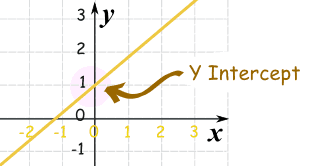# Slope Intercept Form

Standard

### y = mx + b

m = Slope (how steep the line is)

b = the Y Intercept (where the line crosses the Y axis)\### How do you find “m” and “b“?

• b is easy: just see where the line crosses the Y axis.
• m (the Slope) needs some calculation. Remember we like to write slope like a fraction.

## Example 1)The fastest and easiest thing to find first when looking at a graph is the y-intercept (b).

Here we see that the line crosses the y-axis at positive 1.

So, b = 1 .

Now to find the slope (m) we will use rise over run:

• Rise is how far up
• Run is how far along

In this example the rise is 2 and the run is 1.

So, m = 2/1 .

Now that I know m = 2/1 and b = 1 I can plug them into the equation for slope intercept form y = mx + b.

y = (2/1) x + 1

^^^^^ This is the equation of the line.

# Y – intercept

Standard

## Y Intercept

Where a straight line crosses the Y axis of a graph.

### Example:In the above diagram the line crosses the Y axis at 1.

So the Y intercept is equal to 1.